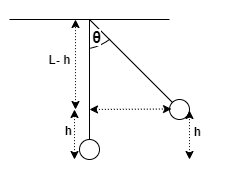# Problem: Suppose a pendulum bob is suspended from a pivot by a massless string of length L, measured to the center of the bob.a) Show that if I draw the pendulum back by an angle θ, the center of the bob is elevated by the distance     delta (h) = L(1-cosθ) = 2Lsin^2(θ/2)b)Using the results of part (a), show that the speed of the pendulum bob at the bottom of its travel should be   v = 2√gl * sin(θ/2)

###### FREE Expert Solution

We're given that the length of the string is L.

The change in height is Δh

a)

Let's consider Δh to be the height that the bob rises when it makes an angle of θ with the vertical.

Diagrammatically, we have:80% (69 ratings)###### Problem Details

Suppose a pendulum bob is suspended from a pivot by a massless string of length L, measured to the center of the bob.
a) Show that if I draw the pendulum back by an angle θ, the center of the bob is elevated by the distance

delta (h) = L(1-cosθ) = 2Lsin^2(θ/2)

b)Using the results of part (a), show that the speed of the pendulum bob at the bottom of its travel should be

v = 2√gl * sin(θ/2)